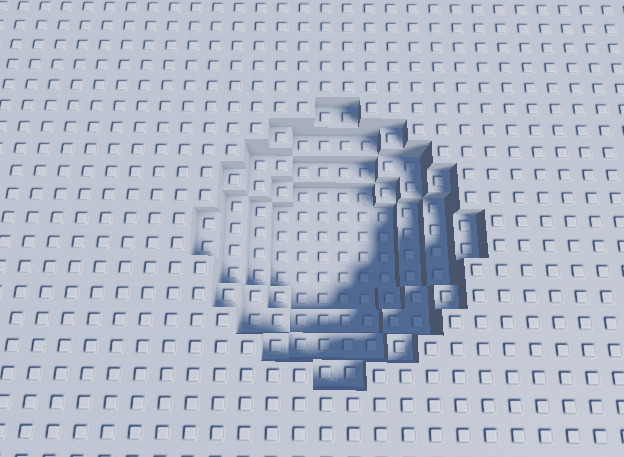# How would I find the last coordinate to fulfil this condition?

Given a Position, a Distance and another Vector3 missing one of its coordinates, how would I be able to calculate with a function the missing coordinate necessary to make this Vector3 and the Position have said Distance to each other?

As a visual representation, I want to calculate ‘?’ in this picture.

What exactly are you trying to create, with the lack of that information its kind of hard to interpret what your looking for

After an explosion, I want to create a crater. This being said, I want to find out, for a certain Vector3 (x, ?, z), what the ? coordinate is for those values in x and y, so the vector is on the surface of the sphere with radius = distance. Also, I would really rather not use Raycasting to solve this problem.

Wouldn’t you have to take into account the explosion size too?

Yes. Details such as position and Radius are being passed as arguments.

So your to simulate the math for a real crater is what im understanding?

Yes, that would be an appropriate description. I presented the problem in a mostly math focused way.

@MrAfoba
I feel like this is a little bit overkill, since you know the size of the explosion you could just create a crater yourself then size and position it accordingly depending on the factors you talked about above

Unions do not quite work here, if that is what you are thinking. Multiple cuts on a single union bring some performance issues. It is not an option for me.

I was talking about an actual model of a crater that you could place, if your using terrain you could remove the area around it using the explosion’s size data

I am not using terrain. Placing a model would not work cuz the explosion can happen in the edge of a part. I am not really looking for alternative solutions, I want to accomplish what I stated.

1 Like

Still a little confused by your explanation, but I think what you’re trying to figure out is this: given two positions of distance d (representing a radius of a sphere between the two points), with one of them missing a coordinate (y) and the other one being complete, you want to figure out that missing coordinate (y).
If this is the case, you can use geometry for this.
Let’s say the origin position (the vector you know all of the coordinates for) is VectorA, and the radius position is VectorB. VectorA’s coordinates are (a, b, c), and VectorB’s coordinates are (x, y, z), respectively. We will call the radius r.
With all of that information, we can now use the equation for the sphere:

``````(x-a)^2 + (y-b)^2 + (z-c)^2 = r^2
``````

Since we know x, a, b, z, c, and r, we can simply solve for y using algebraic rules yielding the equation:

``````y = SQUAREROOTOF(r^2 - (x-a)^2 - (z-c)^2) + b
``````

Let’s try an example.
The origin of the first sphere is at (2, 2, 2). The second position is at (2, y, 3). The radius is 2.
If we plug values into the equation:

``````y = SQUAREROOTOF(2^2 - (2-2)^2 - (3-2)^2) + 2
``````

This would yield two values of ~ 0.27, and 3.73.
Getting 2 y values makes sense because as seen by your picture in the original post, the line you drew goes through the circle twice, implying two values of y satisfy the equation.

Hopefully I answered your question and this made sense. Also, I may have messed up on the math along the way, so if there are any calculation errors, let me know! The equations should be correct, though.

Also, keep in mind that the radius value MUST be a correct size that will satisfy the x and z coordinates, or else this won’t work. For example, if you have x and z offset values of 200 and 400 respectively, you can’t have a radius value of 1.

1 Like

Yes this is definitely it. I was not aware of the sphere equation either. Thank you very much!1 Like

Yup! I just modified the algebraic equation for a circle into a 3 dimensional version. If you have any further questions about this topic, feel free to ask. Or look them up on the internet. There are tons of resources on sphere algebra on google, I’m sure.

I will try to give it a shot really quick and will give some feedback afterwards!

1 Like

@xendatro It is working wonders! Once more, thank you so much for your help!